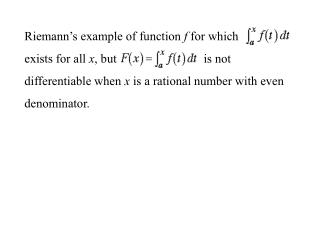DownloadDownload PresentationRiemann’s example of function f for which exists for all x , but is not

# Riemann’s example of function f for which exists for all x , but is not

Télécharger la présentation## Riemann’s example of function f for which exists for all x , but is not

- - - - - - - - - - - - - - - - - - - - - - - - - - - E N D - - - - - - - - - - - - - - - - - - - - - - - - - - -
##### Presentation Transcript

1. Riemann’s example of function f for which exists for all x, but is not differentiable when x is a rational number with even denominator.

2. Riemann’s example of function f for which exists for all x, but is not differentiable when x is a rational number with even denominator. What does a derivative look like? Can we find a function that can’t be a derivative but which can be integrated? Does a derivative have to be continuous?

3. If F is differentiable at x = a, can F '(x) be discontinuous at x = a?

4. Yes! If F is differentiable at x = a, can F '(x) be discontinuous at x = a?

5. How discontinuous can a derivative be? Can it have jump discontinuities where the limits from left and right exist, but are not equal?

6. No! How discontinuous can a derivative be? Can it have jump discontinuities where the limits from left and right exist, but are not equal?

7. The derivative of a function cannot have any jump discontinuities!

8. Bernhard Riemann (1852, 1867) On the representation of a function as a trigonometric series Defined as limit of

9. Bernhard Riemann (1852, 1867) On the representation of a function as a trigonometric series Defined as limit of Key to convergence: on each interval, look at the variation of the function

10. Integral exists if and only if can be made as small as we wish by taking sufficiently small intervals. Bernhard Riemann (1852, 1867) On the representation of a function as a trigonometric series Defined as limit of Key to convergence: on each interval, look at the variation of the function

11. Any continuous function is integrable:

12. Bernhard Riemann (1852, 1867) On the representation of a function as a trigonometric series Riemann gave an example of a function that has a jump discontinuity in every subinterval of [0,1], but which can be integrated over the interval [0,1].

13. –2 –1 1 2 Riemann’s function:

14. At the function jumps by Riemann’s function: The key to the integrability is that given any positive number, no matter how small, there are only a finite number of places where is jump is larger than that number.

15. Riemann’s function: At the function jumps by The key to the integrability is that given any positive number, no matter how small, there are only a finite number of places where is jump is larger than that number. Conclusion: